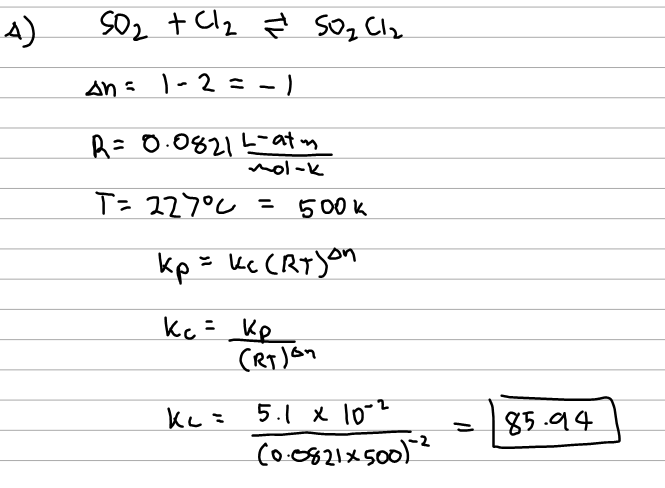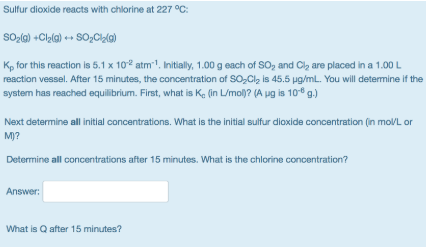# Problem: Sulfur dioxide reacts with chlorine at 227°C: SO2(g) +Cl2(g) &lt;—&gt; SO2Cl2(g) Kp for this reaction is 5.1 x 10-2 atm-1. Initially, 1.00 g each of SO2 and Cl2 are placed in a 1.00 L reaction vessel. After 15 minutes, the concentration of SO2Cl2 is 45.5 μg/mL. You will determine if the system has reached equilibrium. First, what is Kc, (in L/mol)? (A μg is 10-6 g.) Next determine all initial concentrations. What is the initial sulfur dioxide concentration (in mol/L or M)? Determine all concentrations after 15 minutes. What is the chlorine concentration? What is Q after 15 minutes?

###### FREE Expert Solution83% (170 ratings)###### Problem Details

Sulfur dioxide reacts with chlorine at 227°C:

SO2(g) +Cl2(g) <—> SO2Cl2(g)

Kp for this reaction is 5.1 x 10-2 atm-1. Initially, 1.00 g each of SO2 and Cl2 are placed in a 1.00 L reaction vessel. After 15 minutes, the concentration of SO2Cl2 is 45.5 μg/mL. You will determine if the system has reached equilibrium. First, what is Kc, (in L/mol)? (A μg is 10-6 g.)

Next determine all initial concentrations. What is the initial sulfur dioxide concentration (in mol/L or M)?

Determine all concentrations after 15 minutes. What is the chlorine concentration?

What is Q after 15 minutes?Frequently Asked Questions

What scientific concept do you need to know in order to solve this problem?

Our tutors have indicated that to solve this problem you will need to apply the The Reaction Quotient concept. You can view video lessons to learn The Reaction Quotient. Or if you need more The Reaction Quotient practice, you can also practice The Reaction Quotient practice problems.

What professor is this problem relevant for?

Based on our data, we think this problem is relevant for Professor All Professors' class at New Jersey Institute of Technology.